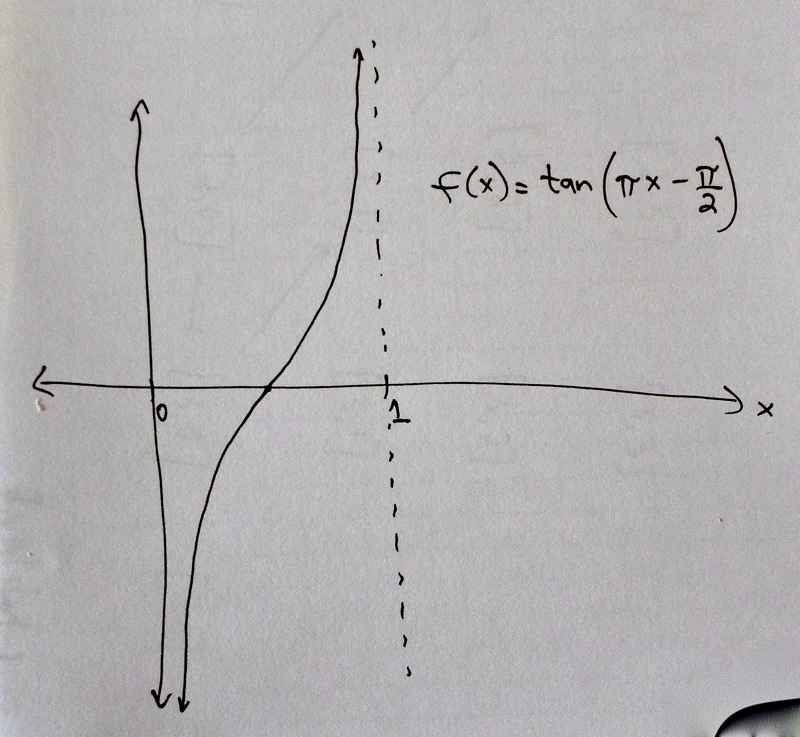# In-Depth

A complete explanation of why $$|\mathbb{R}| = |(0,1)|.$$

To show $$(0,1)$$ and $$\mathbb{R}$$ are the same size, we need a one-to-one correspondence between them. In this case, we can describe the one-to-one correspondence using a function. There are several functions that would work, but we’ll choose one with a nice graph.

Consider the function from $$(0,1)$$ to $$\mathbb{R}$$ defined by $$f(x) = \tan(\pi x - \frac{\pi}{2})$$ for every element $$x$$ in $$(0,1)$$.

Here "tan" means the tangent function, from trigonometry. The graph of this function is the following.Now you can see why we wanted to drop $$t=0$$ and $$t=1$$ from our set corresponding to breathed infinity: the function $$f$$ above is undefined at $$t=0$$ and $$t=1$$.

Next we must convince ourselves that this is a one-to-one correspondence. It is defined on the interval $$(0,1)$$; in other words, it sends every element of $$(0,1)$$ somewhere. More specifically, it sends every element of $$(0,1)$$ to some real number.

Furthermore, this function $$f$$ sends every element of $$(0,1)$$ to a different real number. No two elements of $$(0,1)$$ get sent to the same real number. We know this, basically, because of what we know about the tangent function. But this is also easy to see by looking at the graph; the graph of $$f$$ passes the "horizontal line test". For each point on the y-axis, a horizontal line drawn there hits the graph at most once.

(An example that fails the horizontal line test is the function $$g(x) = x^2$$. This is a parabola. Both $$3$$ and $$-3$$ get sent to $$9$$. In other words, $$g(3) = g(-3) = 9$$. This function $$g$$ is sort of "two-to-one", whereas $$f$$ is "one-to-one.")

The last thing we need to check is that every element of $$\mathbb{R}$$ gets hit by some element of $$(0,1)$$, using the function $$f$$. The graph of $$f$$ goes up higher and higher as the input moves closer to $$1$$. The tangent function $$\tan(x)$$ has a vertical asymptote at $$\frac{\pi}{2}$$ (because tangent is $$\sin x\, / \cos x$$, and $$\cos x$$ approaches zero as $$x$$ approaches $$\frac{\pi}{2}$$.) The function $$f$$ is a shift and compression of $$\tan(x)$$, so it has a vertical asymptote at $$t=1$$.

This means that $$f(x)$$ does in fact get arbitrarily high, as $$x$$ approaches $$1$$. For any positive real number $$z$$, no matter how large, on the y-axis, if we draw a horizontal line there, the graph will reach up to cross it. In other words, there is some number $$w$$ in $$(0,1)$$ so that $$f(w) = z$$.

The same is true for negative real numbers: the function $$f$$ goes arbitrarily far down as its input approaches $$0$$. So we conclude that for every number $$z$$ in $$\mathbb{R}$$, there is one and exactly one $$w$$ in $$(0,1)$$ such that $$f(w) = z$$. Therefore $$f$$ is a one-to-one correspondence between $$(0,1)$$ and $$\mathbb{R}$$.

Top